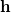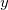Q-Chem 5.0 User’s Manual

# A.1 Introduction

Geometry optimization refers to the determination of stationary points, principally minima and transition states, on molecular potential energy surfaces. It is an iterative process, requiring the repeated calculation of energies, gradients and (possibly) Hessians at each optimization cycle until convergence is attained. The optimization step involves modifying the current geometry, utilizing current and previous energy, gradient and Hessian information to produce a revised geometry which is closer to the target stationary point than its predecessor was. The art of geometry optimization lies in calculating the step, the displacement from the starting geometry on that cycle, so as to converge in as few cycles as possible.

There are four main factors that influence the rate of convergence. These are:

• Initial starting geometry.

• Algorithm used to determine the step.

• Quality of the Hessian (second derivative) matrix.

• Coordinate system chosen.

The first of these factors is obvious: the closer the initial geometry is to the final converged geometry the fewer optimization cycles it will take to reach it. The second factor is again obvious: if a poor stepis predicted, this will obviously slow down the rate of convergence. The third factor is related to the second: the best algorithms make use of second derivative (curvature) information in calculating, and the better this information is, the better will be the predicted step. The importance of the fourth factor (the coordinate system) has been generally appreciated later on: a good choice of coordinates can enhance the convergence rate by an order of magnitude (a factor of 10) or more, depending on the molecule being optimized.

Q-Chem includes a powerful suite of algorithms for geometry optimization written by Jon Baker and known collectively as Optimize. These algorithms have been developed and perfected over the past ten years and the code is robust and has been well tested. Optimize is a general geometry optimization package for locating both minima and transition states. It can optimize using Cartesian, Z-matrix coordinates or delocalized internal coordinates. The last of these are generated automatically from the Cartesian coordinates and are often found to be particularly effective. It also handles fixed constraints on distances, angles, torsions and out-of-plane bends, between any atoms in the molecule, whether or not the desired constraint is satisfied in the starting geometry. Finally it can freeze atomic positions, or any,,Cartesian atomic coordinates.

Optimize is designed to operate with minimal user input. All that is required is the initial guess geometry, either in Cartesian coordinates (e.g., from a suitable model builder such as HyperChem) or as a Z-matrix, the type of stationary point being sought (minimum or transition state) and details of any imposed constraints. All decisions as to the optimization strategy (what algorithm to use, what coordinate system to choose, how to handle the constraints) are made by Optimize.

Note particularly, that although the starting geometry is input in a particular coordinate system (as a Z-matrix, for example) these coordinates are not necessarily used during the actual optimization. The best coordinates for the majority of geometry optimizations are delocalized internals, and these will be tried first. Only if delocalized internals fail for some reason, or if conditions prevent them being used (e.g., frozen atoms) will other coordinate systems be tried. If all else fails the default is to switch to Cartesian coordinates. Similar defaults hold for the optimization algorithm, maximum step size, convergence criteria, etc. You may of course override the default choices and force a particular optimization strategy, but it is not normally necessary to provide Optimize with anything other than the minimal information outlined above.

The heart of the Optimize package (for both minima and transition states) is Baker’s eigenvector-following (EF) algorithm . This was developed following the work of Cerjan and Miller  and Simons and co-workers [897, 898]. The Hessian mode-following option incorporated into this algorithm is capable of locating transition states by walking uphill from the associated minima. By following the lowest Hessian mode, the EF algorithm can locate transition states starting from any reasonable input geometry and Hessian.

An additional option available for minimization is Pulay’s GDIIS algorithm , which is based on the well known DIIS technique for accelerating SCF convergence . GDIIS must be specifically requested, as the EF algorithm is the default.

Although optimizations can be carried out in Cartesian or Z-matrix coordinates, the best choice, as noted above, is usually delocalized internal coordinates. These coordinates were developed by Baker et al. , and can be considered as a further extension of the natural internal coordinates developed by Pulay et al. [900, 545] and the redundant optimization method of Pulay and Fogarasi .

Optimize incorporates a very accurate and efficient Lagrange multiplier algorithm for constrained optimization. This was originally developed for use with Cartesian coordinates [902, 903] and can handle constraints that are not satisfied in the starting geometry. The Lagrange multiplier approach has been modified for use with delocalized internals ; this is much more efficient and is now the default. The Lagrange multiplier code can locate constrained transition states as well as minima.